# Mixed-volume theory

A branch of the theory of convex bodies concerned with the functionals that arise in the study of linear combinations of bodies (see Addition of sets).

The volumeof a linear combinationof convex bodies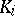in a Euclidean spacewith coefficients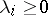is a homogeneous polynomial of degreein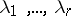: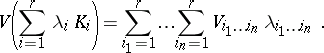(*)

The coefficients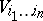are assumed to be symmetric with respect to permutations of the subscripts and are denoted by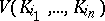, since they depend only on the bodies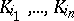. These coefficients are called the mixed volumes of the bodies.

The significance of this theory lies in the universality of the concept of mixed volumes: by substituting concrete bodies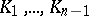into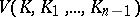, one can obtain various quantities related to a body. These include: its volume, its surface area, the surface integral of the elementary symmetric function of its principal curvatures (in the case of a-smooth body), and also the corresponding characteristics of its projections to-dimensional planes,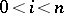. A special case of the expression (*) is the Steiner formula for volumes of parallel bodies in:whereis the volume,the surface area,the total mean curvature of the original body, andthe volume of an-neighbourhood of it. The mixed volumeis invariant under parallel translation of any, is monotonic (with respect to inclusion of bodies), continuous and non-negative;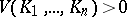if and only if it is possible to choose a segment in everysuch that these segments are linearly independent (see ).

If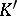is the projection ofto a hypersurface orthogonal to a segmentof length one, then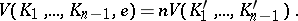The volume of the projection ofto a-dimensional subspace is called its-th cross-sectional measure (or-quermass). Establishing relations between the mean valuesof these measures is one of the concerns of integral geometry. Up to a multiplicative constant, the functionals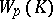coincide with the-th integral curvatures: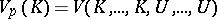(occurrences of,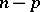occurrences of), whereis the unit sphere. For a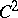-smooth strictly convex body, the mixed volume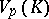,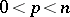, is equal to the integral of the-th elementary symmetric functionof the principal radii of curvature, regarded as a function of the normal to the sphere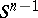. In the case of a general convex body,is the total value of the measureon, defined below and called the curvature function. (In the smooth case,is the density of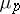.) Just as the volume of the bodyis-th of the integral of its support function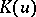with respect to its surface function, i.e. the surface area of the image onunder the spherical mapping (cf. Spherical map), the mixed volume ofbodies can be written as the integral of the support function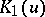of one of them with respect to some measure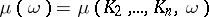onthat depends on the other bodies, called the mixed surface function of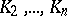:The curvature function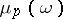is defined by the equation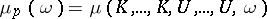(occurrences of,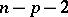occurrences of).

The main content of the theory of mixed volumes is formed by inequalities between mixed volumes (see , ). They include the Minkowski inequality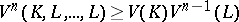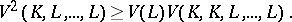Both of these are closely connected with the Brunn–Minkowski theorem, which is true not only for convex bodies. The Aleksandrov–Fenchel inequality generalizes these, and has the following modification (see ):In particular,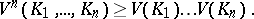A complete system of inequalities characterizing the mixed volume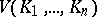has been obtained in the case of two bodies, and more general inequalities have been established (see ).

Many geometric inequalities, such as the classical isoperimetric inequality (cf. Isoperimetric inequality, classical) and several of its refinements, are special cases of inequalities for mixed volumes of convex bodies. The extremum of one of the functionalswhen some other such functional is fixed is attained for a sphere. Inequalities in the theory of mixed volumes are used in proving the uniqueness of the solution of the generalized Minkowski problem (see ), in establishing stability in Minkowski problems (see ) and Weil problems (see ), and in the solution of the van der Waerden problem on permanents (see ). Infinite-dimensional analogues of the concepts of the theory of mixed volumes have found application in the theory of Gaussian stochastic processes (see ).

The theory of mixed volumes has deep connections with algebraic geometry. Given a polynomialincomplex variables, one can define its Newton polyhedron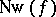in the following way. To each monomial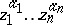occurring inwith a non-zero coefficient, one assigns the point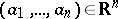, and one definesas the convex hull of these points. The typical number of solutions of the system of polynomial equations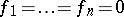is equal to the mixed volume of the polyhedron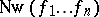divided by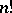. Among other things, this allows one to give an algebraic proof of the Aleksandrov–Fenchel inequality (see ).

In the theory of mixed volumes, a convex body is identified with its support function. This is extended to differences of these functions, and then to arbitrary continuous functions on a sphere (see , ). Using the analogous decomposition of the vector of the centre of gravity of a body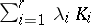multiplied by its volume, one can define the so-called mixed direction vectors, which are vector analogues of mixed volumes. The centres of gravity of a bodycoincide up to a multiplicative constant with the mixed direction vectors ofand the sphere(see ).